Скачать презентацию Konstantinos Makris Electrical Engineering Department Princeton University USA

3b6d72a58b0113c61620c41c148630de.ppt

• Количество слайдов: 35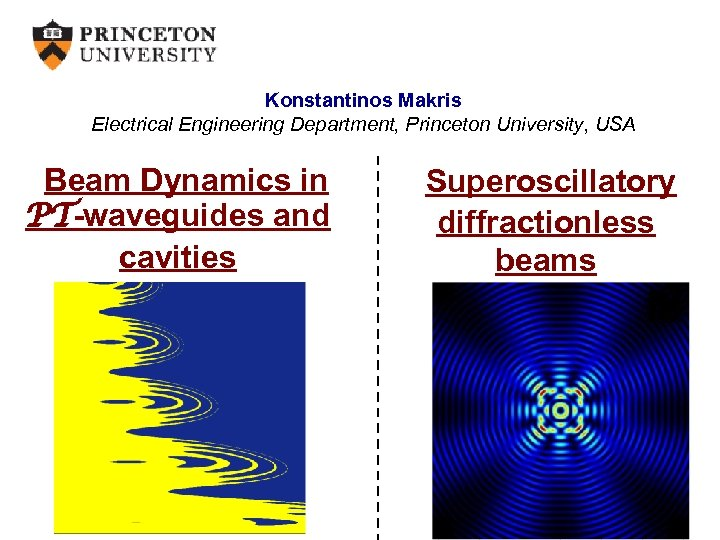Konstantinos Makris Electrical Engineering Department, Princeton University, USA Beam Dynamics in PT-waveguides and cavities Superoscillatory diffractionless beams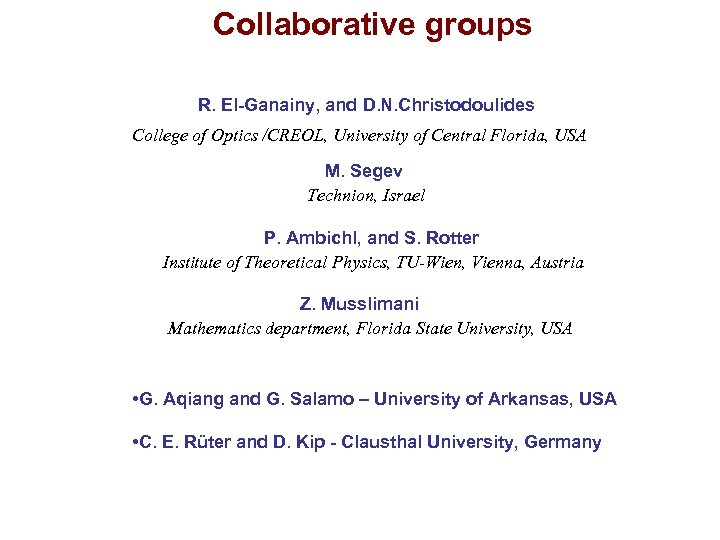Collaborative groups R. El-Ganainy, and D. N. Christodoulides College of Optics /CREOL, University of Central Florida, USA M. Segev Technion, Israel P. Ambichl, and S. Rotter Institute of Theoretical Physics, TU-Wien, Vienna, Austria Z. Musslimani Mathematics department, Florida State University, USA • G. Aqiang and G. Salamo – University of Arkansas, USA • C. E. Rüter and D. Kip - Clausthal University, Germany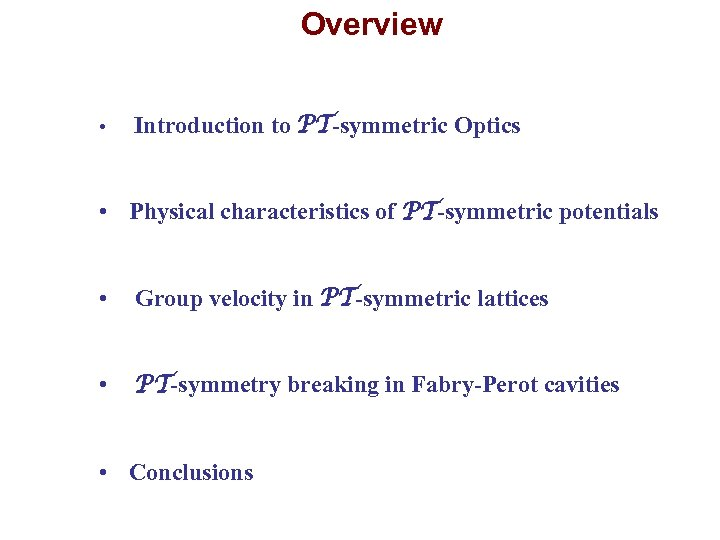Overview • Introduction to PT-symmetric Optics • Physical characteristics of PT-symmetric potentials • Group velocity in PT-symmetric lattices • PT-symmetry breaking in Fabry-Perot cavities • Conclusions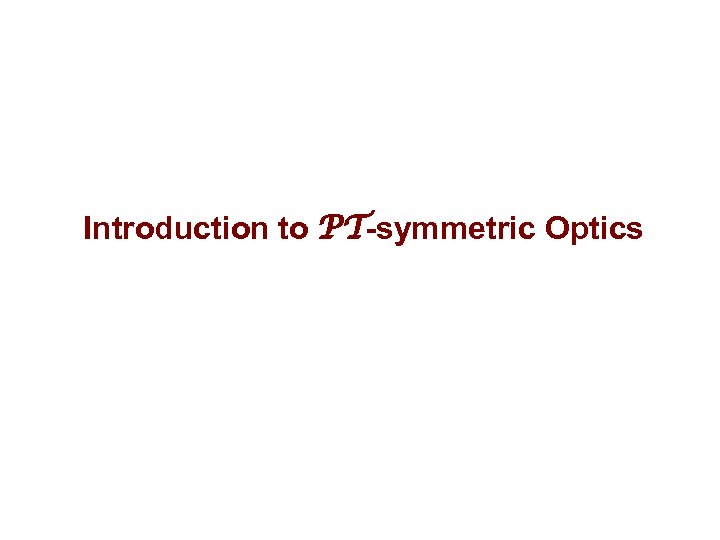Introduction to PT-symmetric Optics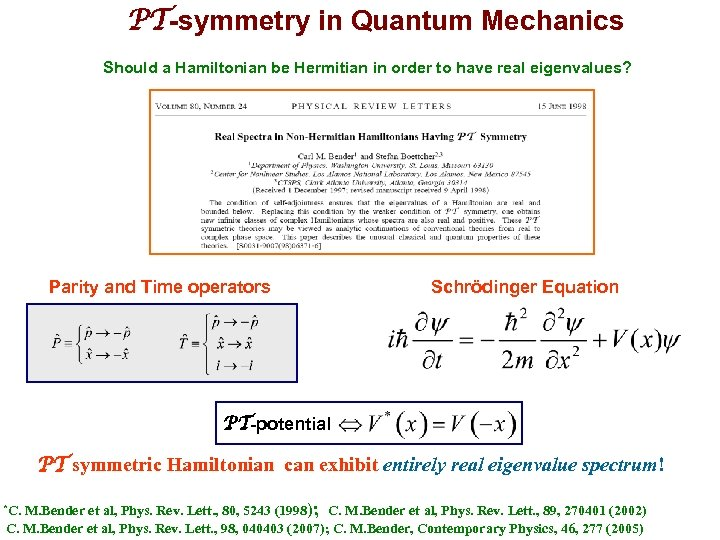PT-symmetry in Quantum Mechanics Should a Hamiltonian be Hermitian in order to have real eigenvalues? Parity and Time operators Schrödinger Equation PT-potential PT symmetric Hamiltonian can exhibit entirely real eigenvalue spectrum! M. Bender et al, Phys. Rev. Lett. , 80, 5243 (1998); C. M. Bender et al, Phys. Rev. Lett. , 89, 270401 (2002) C. M. Bender et al, Phys. Rev. Lett. , 98, 040403 (2007); C. M. Bender, Contemporary Physics, 46, 277 (2005) *C.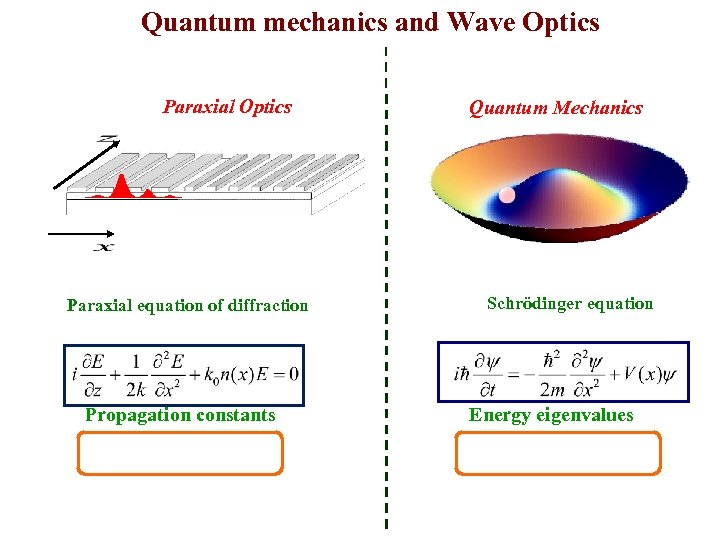Quantum mechanics and Wave Optics Paraxial equation of diffraction Propagation constants Quantum Mechanics Schrödinger equation Energy eigenvalues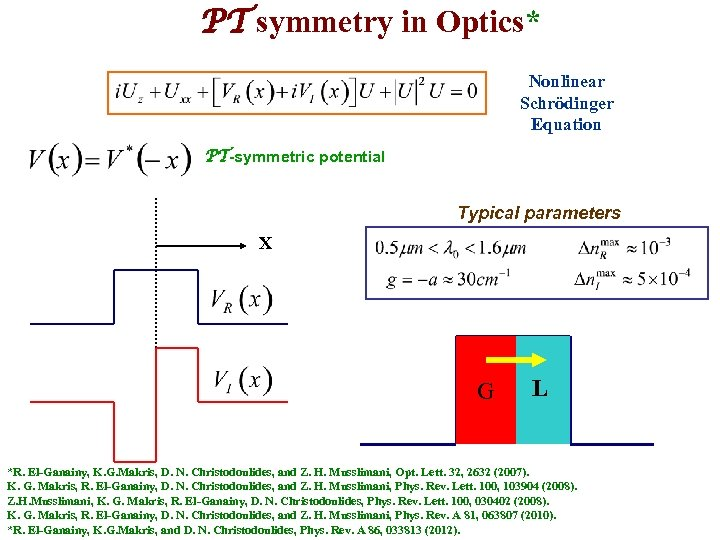PT symmetry in Optics* Nonlinear Schrödinger Equation PT-symmetric potential Typical parameters X G L *R. El-Ganainy, K. G. Makris, D. N. Christodoulides, and Z. H. Musslimani, Opt. Lett. 32, 2632 (2007). K. G. Makris, R. El-Ganainy, D. N. Christodoulides, and Z. H. Musslimani, Phys. Rev. Lett. 100, 103904 (2008). Z. H. Musslimani, K. G. Makris, R. El-Ganainy, D. N. Christodoulides, Phys. Rev. Lett. 100, 030402 (2008). K. G. Makris, R. El-Ganainy, D. N. Christodoulides, and Z. H. Musslimani, Phys. Rev. A 81, 063807 (2010). *R. El-Ganainy, K. G. Makris, and D. N. Christodoulides, Phys. Rev. A 86, 033813 (2012).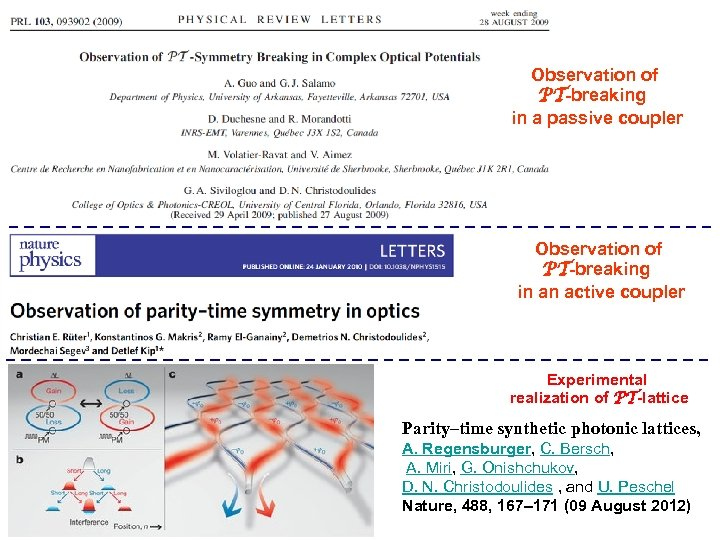Observation of PT-breaking in a passive coupler Observation of PT-breaking in an active coupler Experimental realization of PT-lattice Parity–time synthetic photonic lattices, A. Regensburger, C. Bersch, A. Miri, G. Onishchukov, D. N. Christodoulides , and U. Peschel Nature, 488, 167– 171 (09 August 2012)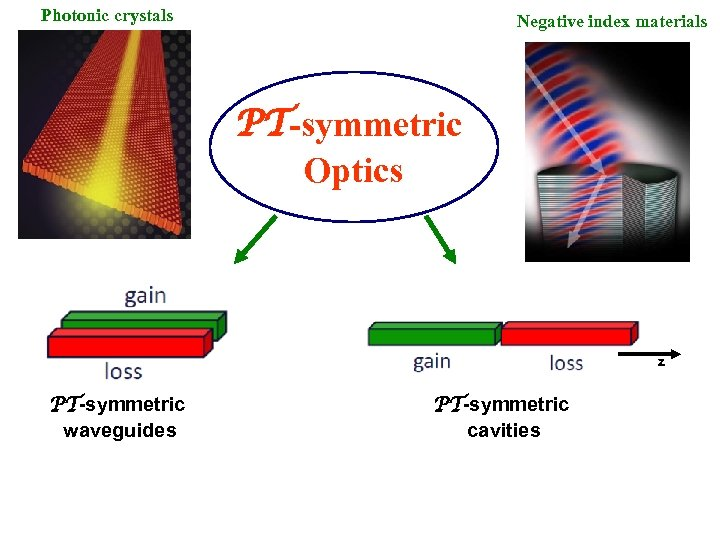Photonic crystals Negative index materials PT-symmetric Optics z PT-symmetric waveguides PT-symmetric cavities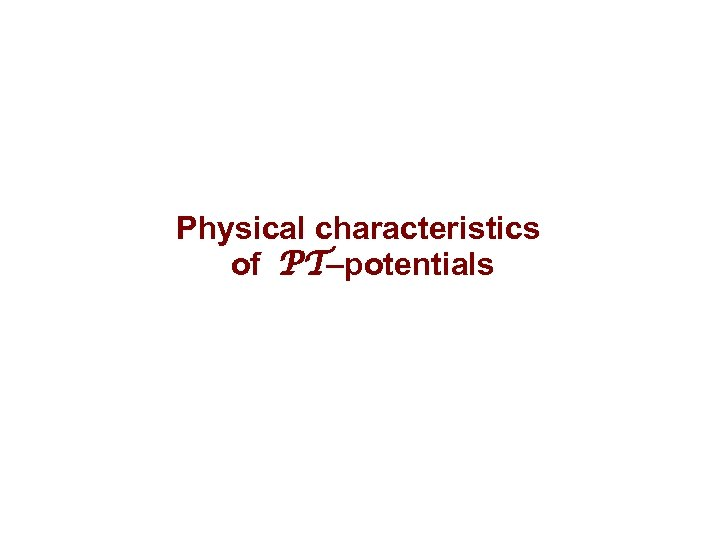Physical characteristics of PT–potentials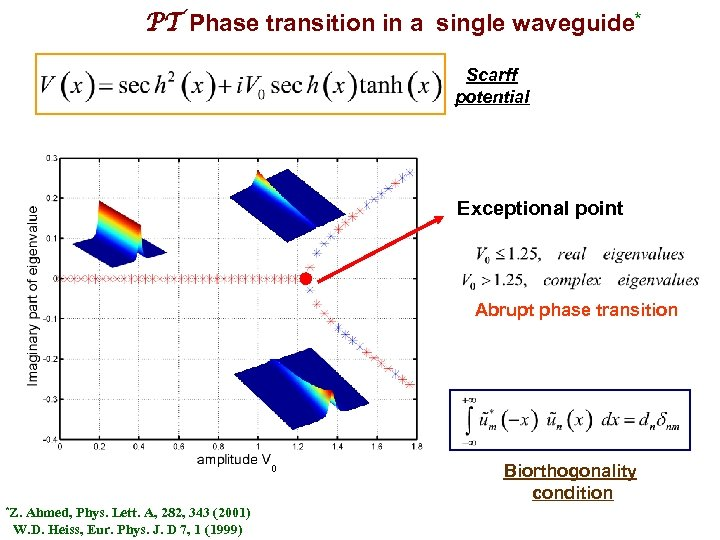PT Phase transition in a single waveguide* Scarff potential Exceptional point Abrupt phase transition 0 *Z. Ahmed, Phys. Lett. A, 282, 343 (2001) W. D. Heiss, Eur. Phys. J. D 7, 1 (1999) Biorthogonality condition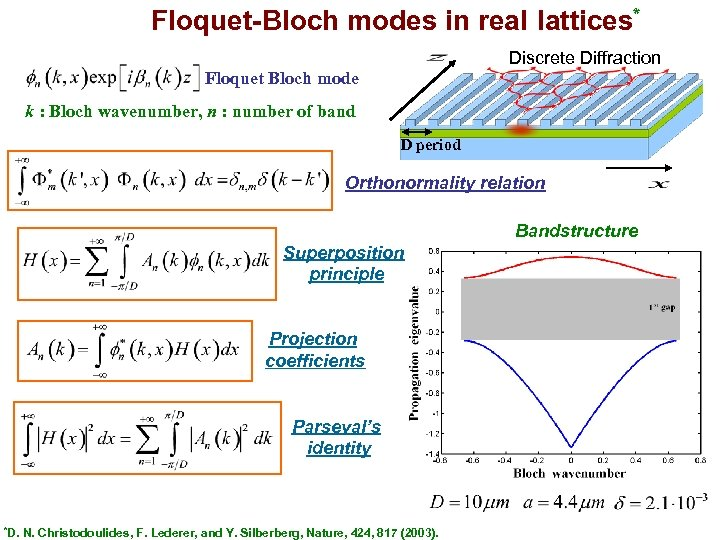Floquet-Bloch modes in real lattices* Discrete Diffraction Floquet Bloch mode k : Bloch wavenumber, n : number of band D period Orthonormality relation Bandstructure Superposition principle Projection coefficients Parseval’s identity *D. N. Christodoulides, F. Lederer, and Y. Silberberg, Nature, 424, 817 (2003).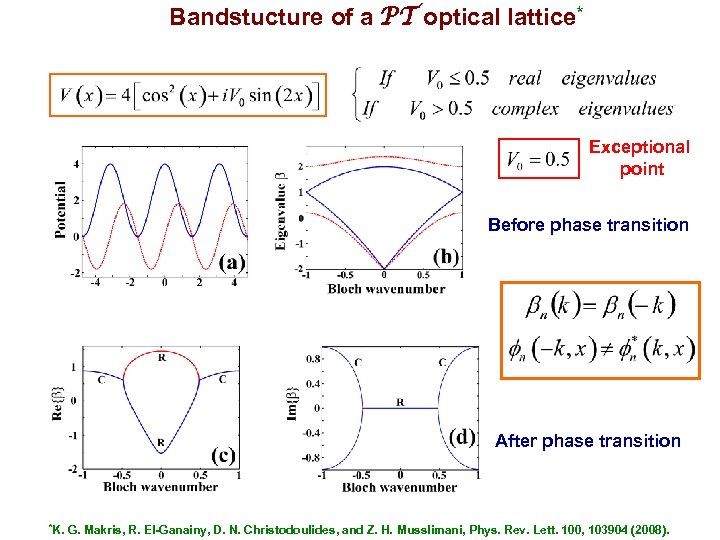Bandstucture of a PT optical lattice* Exceptional point Before phase transition After phase transition *K. G. Makris, R. El-Ganainy, D. N. Christodoulides, and Z. H. Musslimani, Phys. Rev. Lett. 100, 103904 (2008).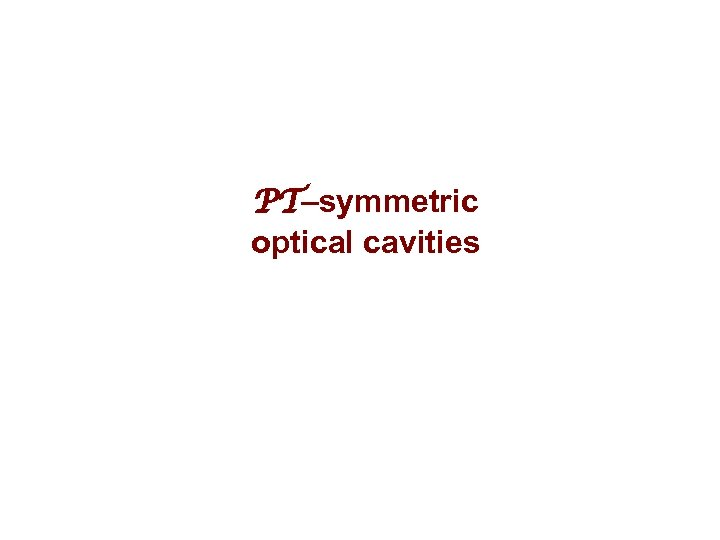PT–symmetric optical cavities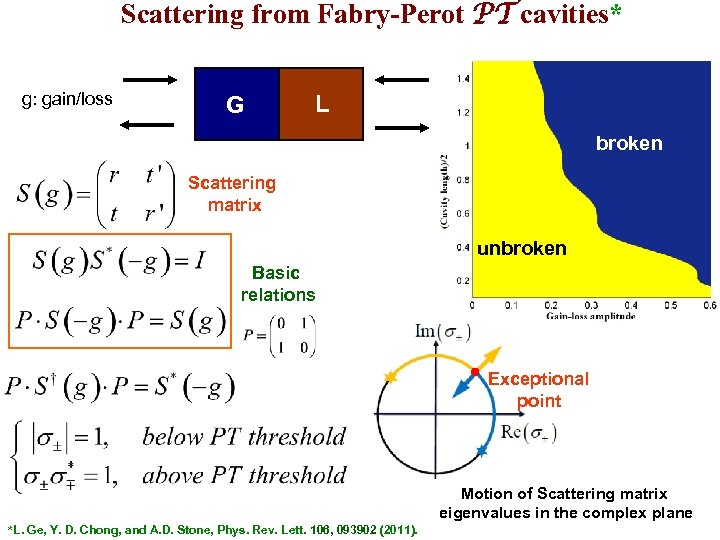Scattering from Fabry-Perot PT cavities* g: gain/loss G L broken Scattering matrix unbroken Basic relations Exceptional point Motion of Scattering matrix eigenvalues in the complex plane *L. Ge, Y. D. Chong, and A. D. Stone, Phys. Rev. Lett. 106, 093902 (2011).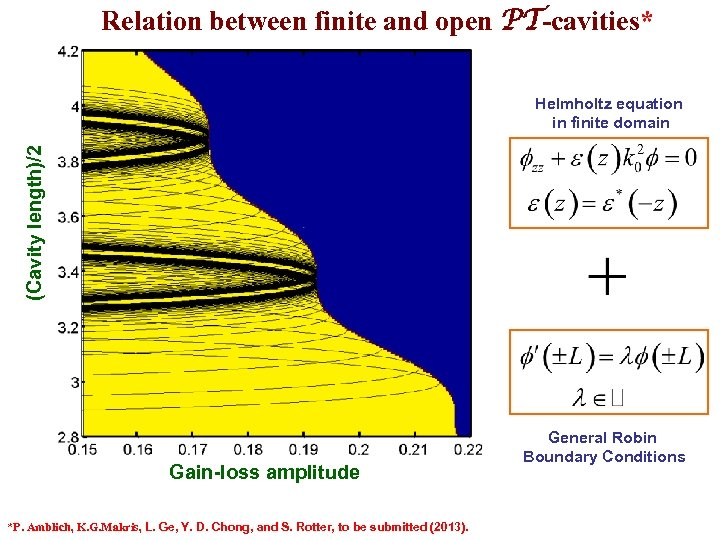Relation between finite and open PT-cavities* (Cavity length)/2 Helmholtz equation in finite domain Gain-loss amplitude *P. Amblich, K. G. Makris, L. Ge, Y. D. Chong, and S. Rotter, to be submitted (2013). General Robin Boundary Conditions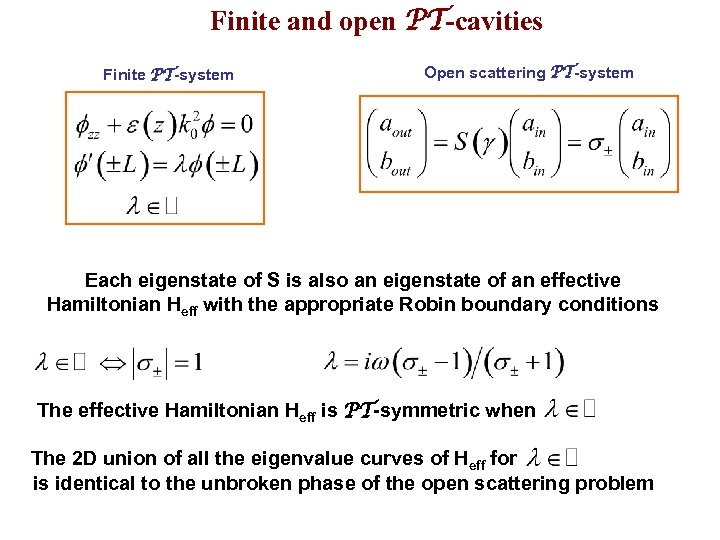Finite and open PT-cavities Finite PT-system Open scattering PT-system Each eigenstate of S is also an eigenstate of an effective Hamiltonian Heff with the appropriate Robin boundary conditions The effective Hamiltonian Heff is PT-symmetric when The 2 D union of all the eigenvalue curves of Heff for is identical to the unbroken phase of the open scattering problem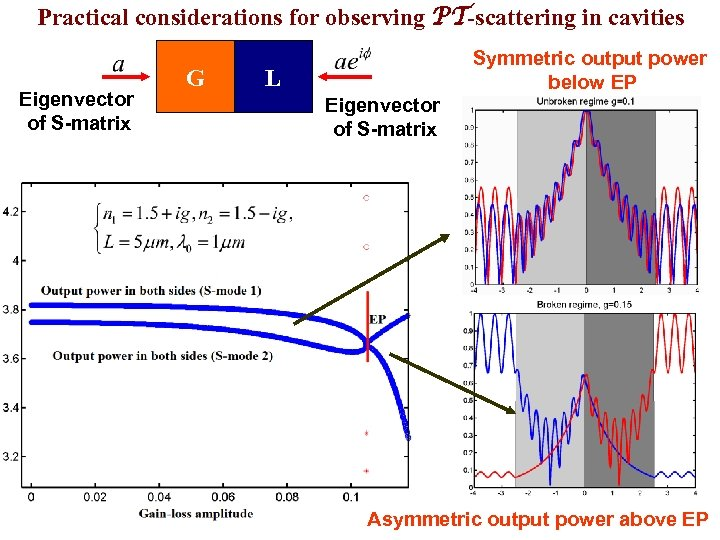Practical considerations for observing PT-scattering in cavities Eigenvector of S-matrix G Symmetric output power below EP L Eigenvector of S-matrix broken Asymmetric output power above EP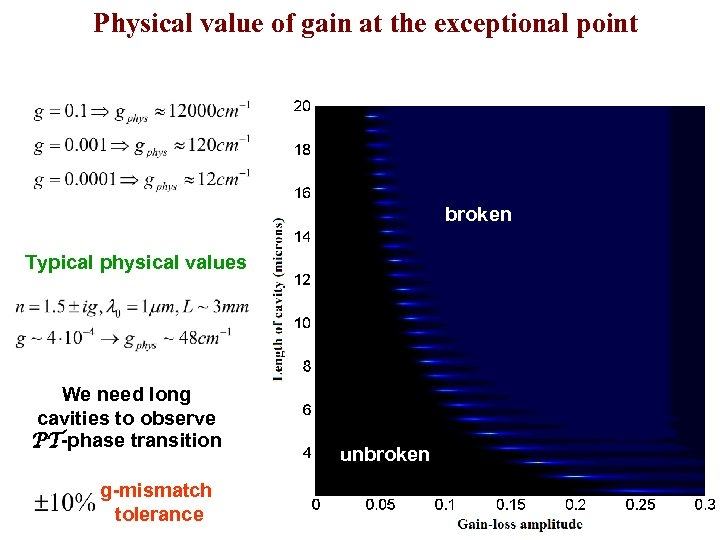Physical value of gain at the exceptional point broken Typical physical values We need long cavities to observe PT-phase transition g-mismatch tolerance unbroken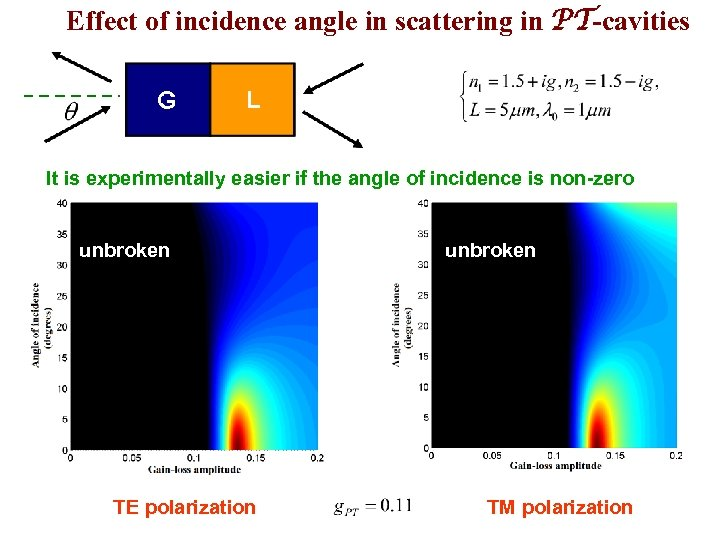Effect of incidence angle in scattering in PT-cavities It is experimentally easier if the angle of incidence is non-zero unbroken TE polarization unbroken TM polarization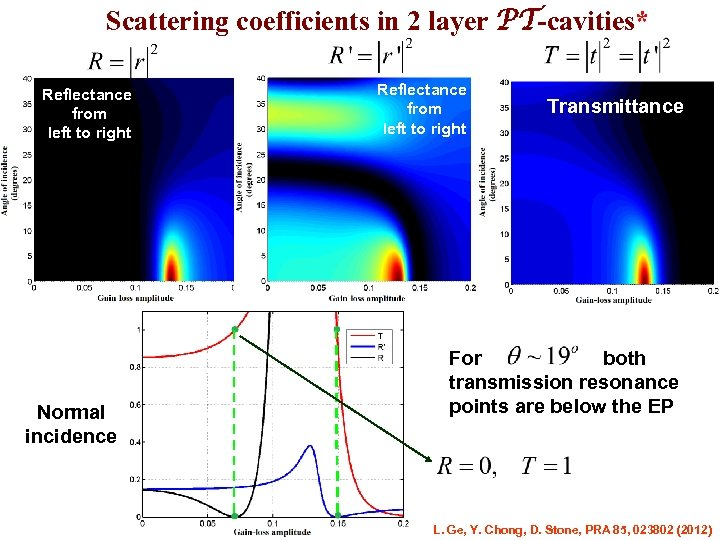Scattering coefficients in 2 layer PT-cavities* Reflectance from left to right Normal incidence Reflectance from left to right Transmittance For both transmission resonance points are below the EP L. Ge, Y. Chong, D. Stone, PRA 85, 023802 (2012)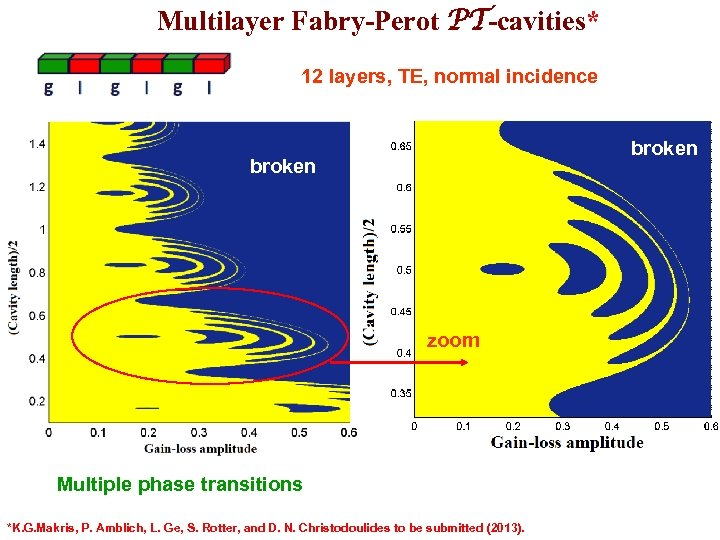Multilayer Fabry-Perot PT-cavities* 12 layers, TE, normal incidence broken zoom Multiple phase transitions *K. G. Makris, P. Amblich, L. Ge, S. Rotter, and D. N. Christodoulides to be submitted (2013).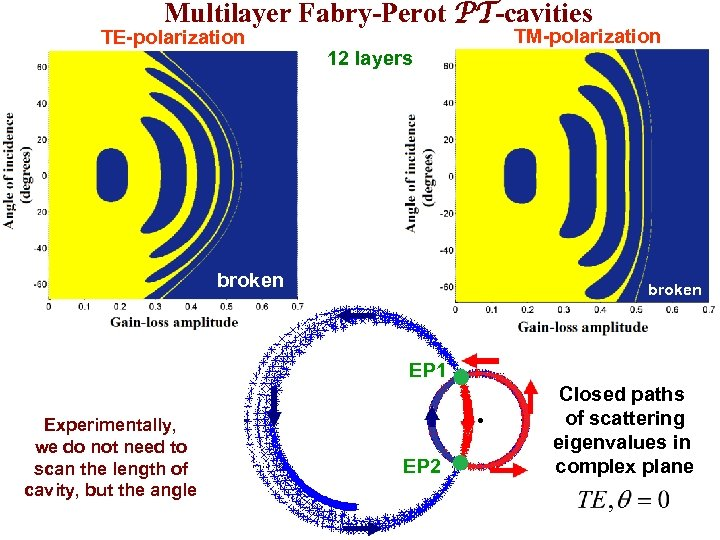Multilayer Fabry-Perot PT-cavities TE-polarization TM-polarization 12 layers broken EP 1 Experimentally, we do not need to scan the length of cavity, but the angle EP 2 Closed paths of scattering eigenvalues in complex plane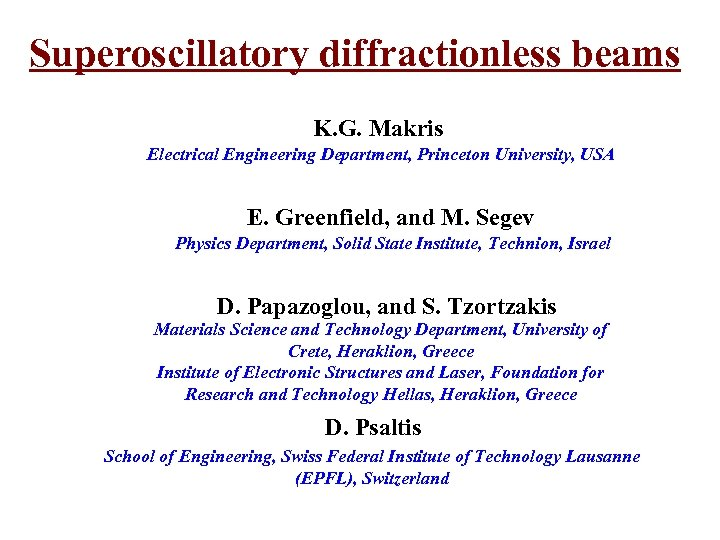Superoscillatory diffractionless beams K. G. Makris Electrical Engineering Department, Princeton University, USA E. Greenfield, and M. Segev Physics Department, Solid State Institute, Technion, Israel D. Papazoglou, and S. Tzortzakis Materials Science and Technology Department, University of Crete, Heraklion, Greece Institute of Electronic Structures and Laser, Foundation for Research and Technology Hellas, Heraklion, Greece D. Psaltis School of Engineering, Swiss Federal Institute of Technology Lausanne (EPFL), Switzerland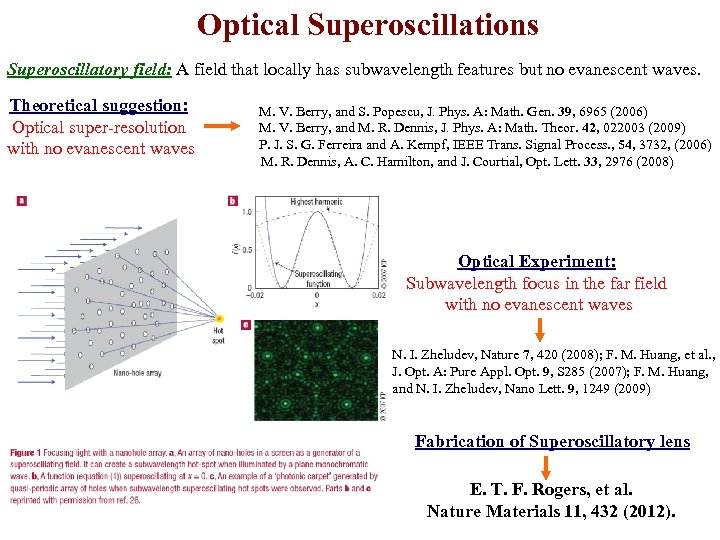Optical Superoscillations Superoscillatory field: A field that locally has subwavelength features but no evanescent waves. Theoretical suggestion: Optical super-resolution with no evanescent waves M. V. Berry, and S. Popescu, J. Phys. A: Math. Gen. 39, 6965 (2006) M. V. Berry, and M. R. Dennis, J. Phys. A: Math. Theor. 42, 022003 (2009) P. J. S. G. Ferreira and A. Kempf, IEEE Trans. Signal Process. , 54, 3732, (2006) M. R. Dennis, A. C. Hamilton, and J. Courtial, Opt. Lett. 33, 2976 (2008) Optical Experiment: Subwavelength focus in the far field with no evanescent waves N. I. Zheludev, Nature 7, 420 (2008); F. M. Huang, et al. , J. Opt. A: Pure Appl. Opt. 9, S 285 (2007); F. M. Huang, and N. I. Zheludev, Nano Lett. 9, 1249 (2009) Fabrication of Superoscillatory lens E. T. F. Rogers, et al. Nature Materials 11, 432 (2012).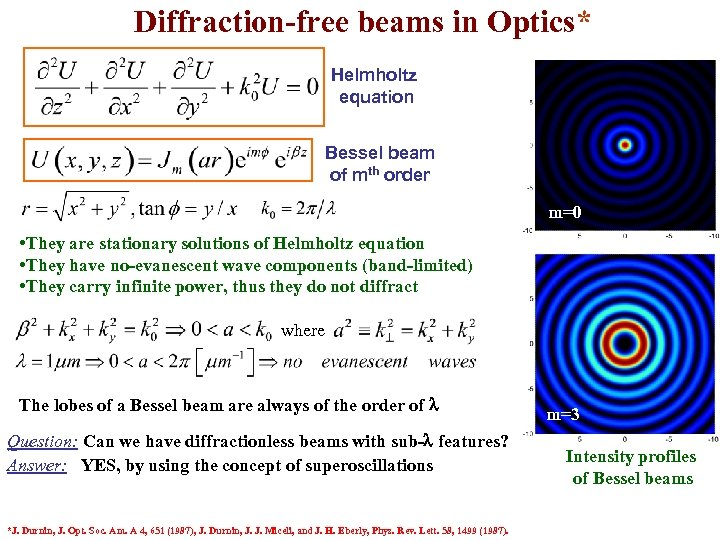Diffraction-free beams in Optics* Helmholtz equation Bessel beam of mth order m=0 • They are stationary solutions of Helmholtz equation • They have no-evanescent wave components (band-limited) • They carry infinite power, thus they do not diffract where The lobes of a Bessel beam are always of the order of l Question: Can we have diffractionless beams with sub-l features? Answer: YES, by using the concept of superoscillations m=3 *J. Durnin, J. Opt. Soc. Am. A 4, 651 (1987), J. Durnin, J. J. Miceli, and J. H. Eberly, Phys. Rev. Lett. 58, 1499 (1987). m=3 Intensity profiles of Bessel beams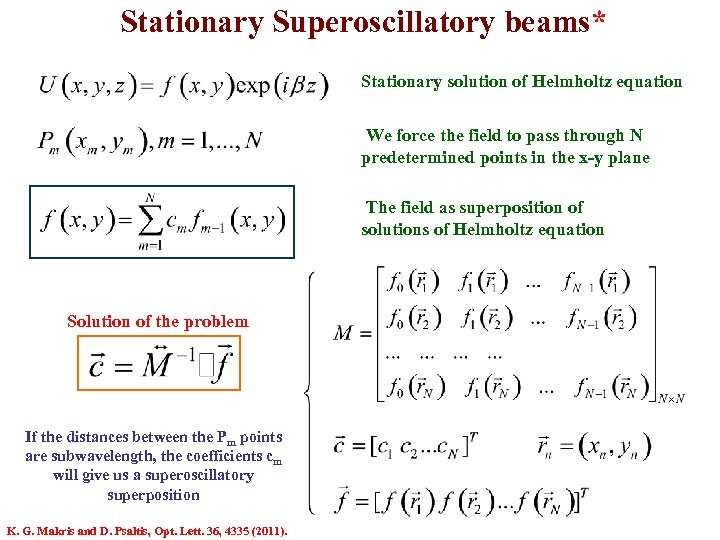Stationary Superoscillatory beams* Stationary solution of Helmholtz equation We force the field to pass through N predetermined points in the x-y plane The field as superposition of solutions of Helmholtz equation Solution of the problem If the distances between the Pm points are subwavelength, the coefficients cm will give us a superoscillatory superposition K. G. Makris and D. Psaltis, Opt. Lett. 36, 4335 (2011).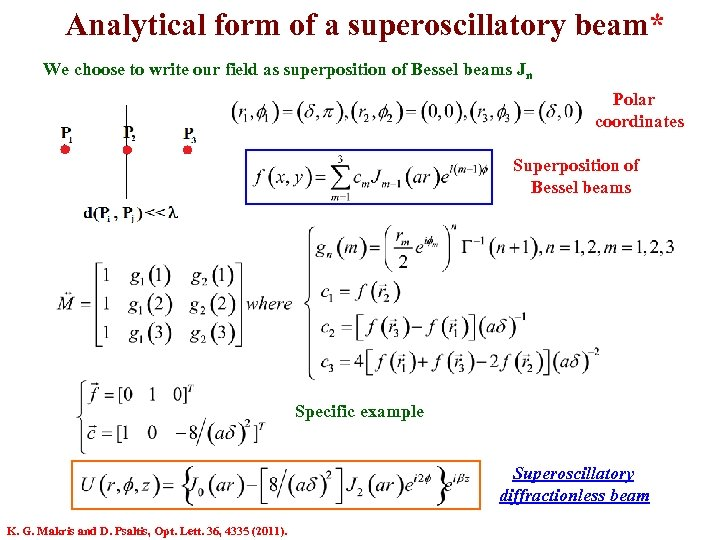Analytical form of a superoscillatory beam* We choose to write our field as superposition of Bessel beams Jn Polar coordinates Superposition of Bessel beams Specific example Superoscillatory diffractionless beam K. G. Makris and D. Psaltis, Opt. Lett. 36, 4335 (2011).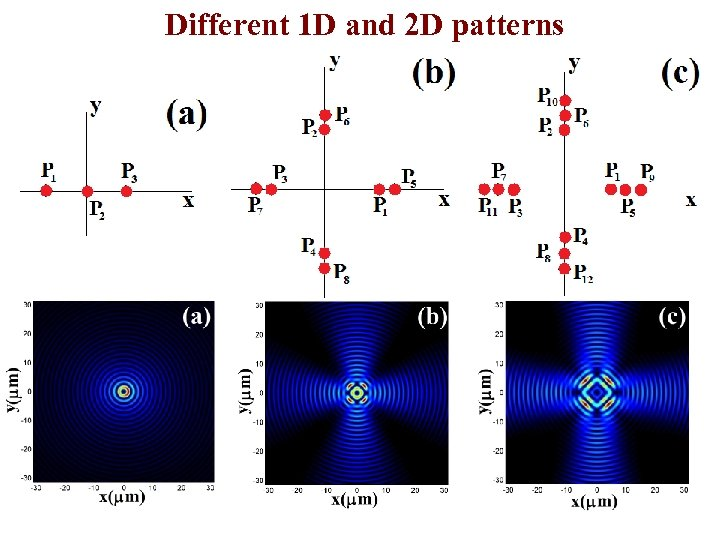Different 1 D and 2 D patterns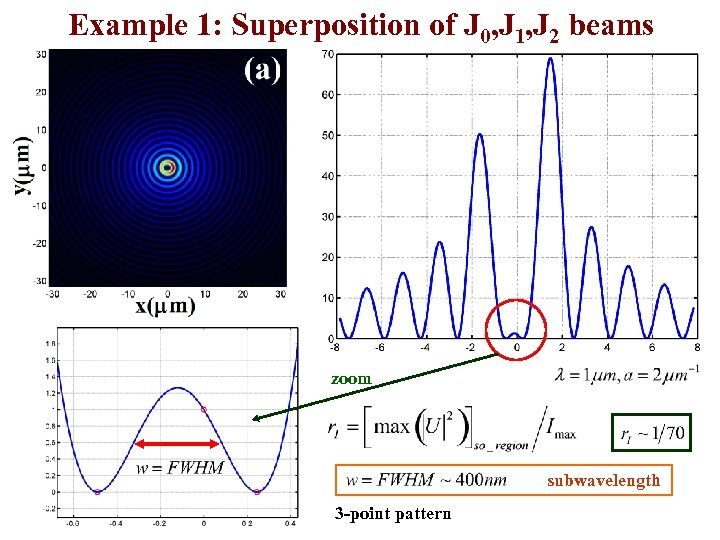Example 1: Superposition of J 0, J 1, J 2 beams zoom subwavelength 3 -point pattern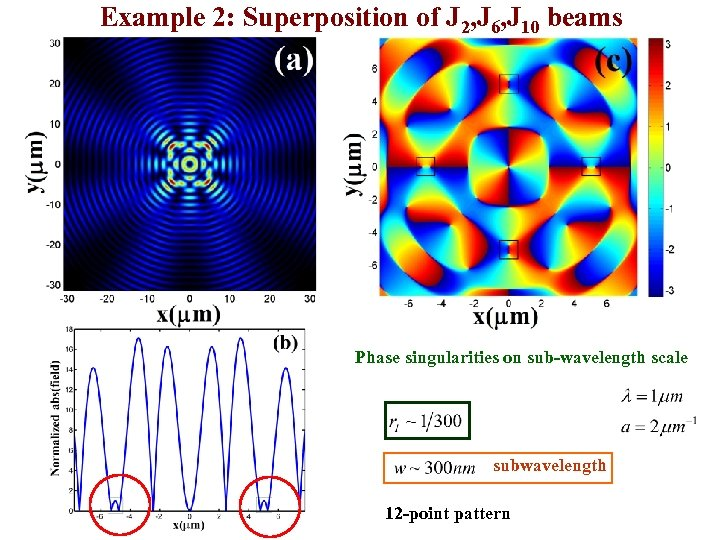Example 2: Superposition of J 2, J 6, J 10 beams Phase singularities on sub-wavelength scale subwavelength 12 -point pattern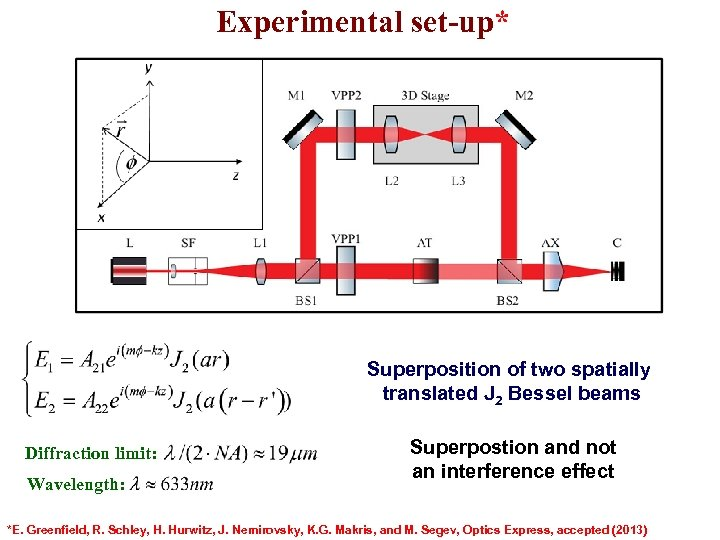Experimental set-up* Superposition of two spatially translated J 2 Bessel beams Diffraction limit: Wavelength: Superpostion and not an interference effect *E. Greenfield, R. Schley, H. Hurwitz, J. Nemirovsky, K. G. Makris, and M. Segev, Optics Express, accepted (2013)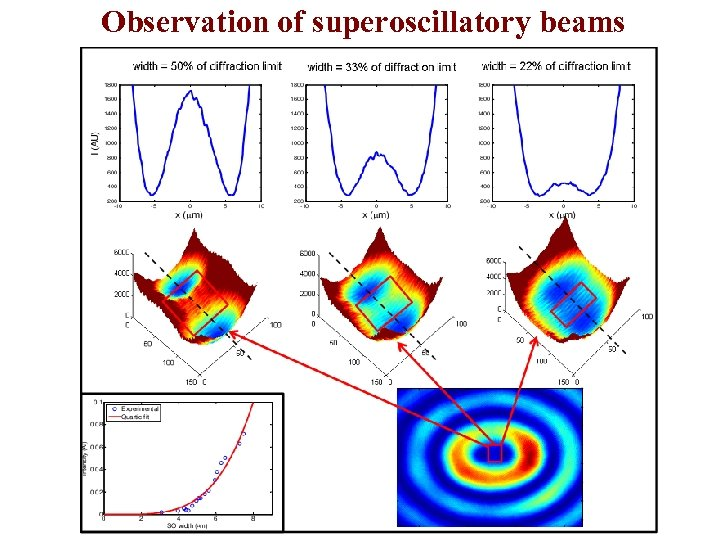Observation of superoscillatory beams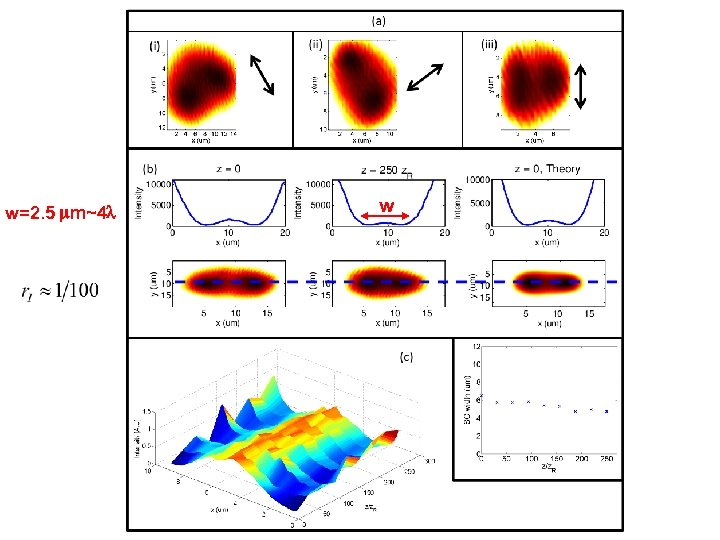w=2. 5 mm~4 l w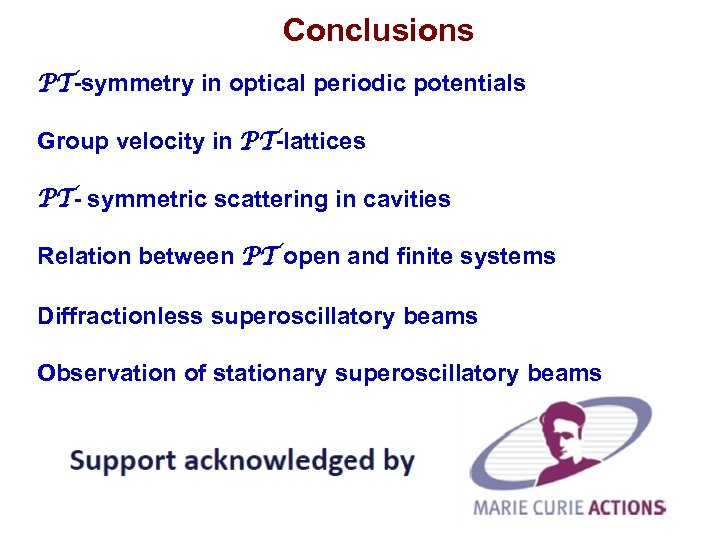Conclusions PT-symmetry in optical periodic potentials Group velocity in PT-lattices PT- symmetric scattering in cavities Relation between PT open and finite systems Diffractionless superoscillatory beams Observation of stationary superoscillatory beams